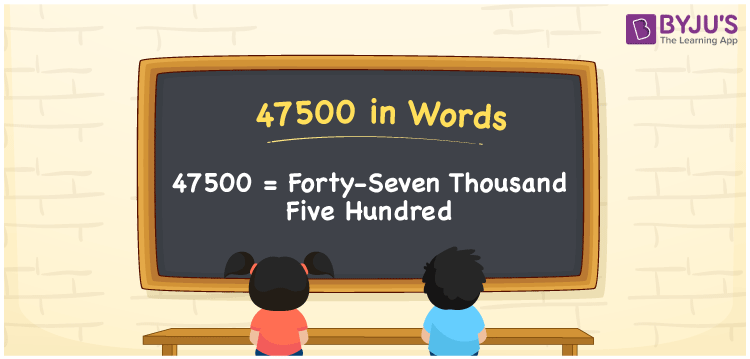# 47500 in Words

47500 in words can be written as Forty-seven Thousand Five Hundred. For example, if you buy a new phone for Rs. 47500 at the store, then you can say that “I bought a new phone for Forty-seven Thousand Five Hundred Rupees at the store”. You will learn in brief regarding the numbers in words with the help of the English alphabets. Therefore, the number 47500 in words can be read as “Forty-seven Thousand Five Hundred”.

 47500 in words Forty-seven Thousand Five Hundred Forty-seven Thousand Five Hundred in Numbers 47500

## 47500 in English Words## How to Write 47500 in Words?

Learn about the place value chart 47500 here. Five digits are present in 47500. The table given will help you know the place value of the five digits in a systematic manner.

 Ten Thousands Thousands Hundreds Tens Ones 4 7 5 0 0

The number 47500 in expanded form is:

4 x Ten Thousand + 7 x Thousand + 5 × Hundred + 0 × Ten + 0 × One

= 4 x 10000 + 7 x 1000 + 5 × 100 + 0 × 10 + 0 × 1

= 40000 + 7000 + 500

= 47500

= Forty-seven Thousand Five Hundred

Therefore, 47500 in words is written as Forty-seven Thousand Five Hundred.

47500 is a natural number that precedes 47501 and succeeds 47499.

47500 in words – Forty-seven Thousand Five Hundred

Is 47500 an odd number? – No

Is 47500 an even number? – Yes

Is 47500 a perfect square number? – No

Is 47500 a perfect cube number? – No

Is 47500 a prime number? – No

Is 47500 a composite number? – Yes

## Frequently Asked Questions on 47500 in Words

Q1

### Write 47500 in words.

47500 can be written as “Forty-seven Thousand Five Hundred” in words.
Q2

### What is the value of 48000 minus 500?

The value of 48000 minus 500 is 47500. Therefore, 47500 in words is Forty-seven Thousand Five Hundred.
Q3

### Is 47500 an odd or even number?

47500 is an even number as it is completely divisible by 2.